# Flexible Pavement ESAL Equation

At first glance, this equation looks quite complex – it is.Where: W equals axle applications inverse of equivalency factors (where W18 = number of 18,000 lb (80 kN) single axle loads) Lx equals axle load being evaluated (kips) L18 equals 18 (standard axle load in kips) L2 equals code for axle configuration 1 = single axle 2 = tandem axle 3 = triple axle (added in the 1986 AASHTO Guide) x = axle load equivalency factor being evaluated s = code for standard axle = 1 (single axle) G equals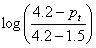a function of the ratio of loss in serviceability at time, t, to the potential loss taken at a point where pt = 1.5 Pt equals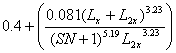"terminal" serviceability index (point at which the pavement is considered to be at the end of its useful life) b equals function which determines the relationship between serviceability and axle load applications SN equals structural number

#### Example Calculation for a Single Axle

• Assumptions:    Single axle, 30,000 lb (133 kN), SN = 3, pt = 2.5
• Answer: (Table D.4, p. D-6, 1993 AASHTO Guide) = 7.9
• Calculationswhere : W18 equals predicted number of 18,000 lb (80 kN) single axle load applications W30 equals predicted number of 30,000 lb (133 kN) single axle load applications Lx equals L30 = 30 L2x equals 1 (single axle) G equals serviceability loss factor equals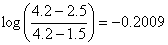b30 equals curve slope factor equals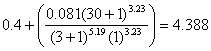and G/b30 equals -0.2009/4.388 = -0.04578 b18 equals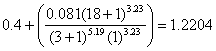G/b18 equals -0.2009/1.2204 = -0.1646 Thus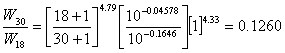and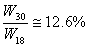Finally LEF equals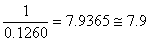(same as contained in 1993 AASHTO Guide, Appendix D)

#### Example Calculation for a Tandem Axle

• Assumptions:    Tandem axle, 40,000 lb (133 kN), SN = 5, pt = 2.5
• Answer: (Table D.5, p. D-7, 1993 AASHTO Guide) = 2.08
• Calculationswhere : L40 equals 40 (tandem axle) L18 equals 18 (single axle) L2x equals 2 (tandem axle) L2s equals 1 (single axle) G equals serviceability loss factor equalsb40 equals curve slope factor equals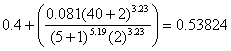and G/b40 equals -0.2009/0.53824 = -0.37325 b18 equals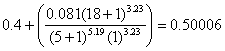G/b18 equals -0.2009/0.50006 = -0.40175 Thus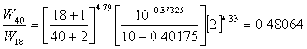Finally LEF equals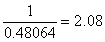(same as contained in 1993 AASHTO Guide nbsp;Appendix D)